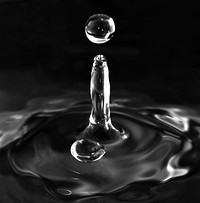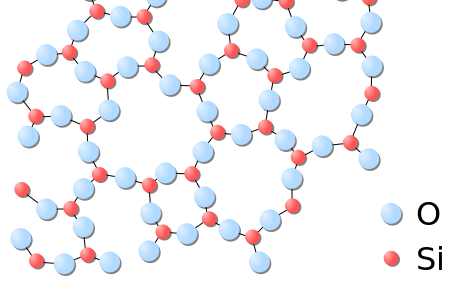# 1.2: Classifying Matter According to Its State: Solid, Liquid, and Gas

Skills to Develop

• To describe the solid, liquid and gas phases.

Water can take many forms. At low temperatures (below $$0^\text{o} \text{C}$$), it is a solid. When at "normal" temperatures (between $$0^\text{o} \text{C}$$ and $$100^\text{o} \text{C}$$), it is a liquid. While at temperatures above $$100^\text{o} \text{C}$$, water is a gas (steam). The state the water is in depends upon the temperature. Each state (solid, liquid, and gas) has its own unique set of physical properties. Matter typically exists in one of three states: solidliquid, or gas.Figure $$\PageIndex{1}$$: Matter is usually classified into three classical states, with plasma sometimes added as a fourth state. From left to right: quartz (solid), water (liquid), nitrogen dioxide (gas).

The state a given substance exhibits is also a physical property. Some substances exist as gases at room temperature (oxygen and carbon dioxide), while others, like water and mercury metal, exist as liquids. Most metals exist as solids at room temperature. All substances can exist in any of these three states. Figure $$\PageIndex{2}$$ shows the differences among solids, liquids, and gases at the molecular level. A solid has definite volume and shape, a liquid has a definite volume but no definite shape, and a gas has neither a definite volume nor shape.Figure $$\PageIndex{2}$$: A Representation of the Solid, Liquid, and Gas States. (a) Solid O2 has a fixed volume and shape, and the molecules are packed tightly together. (b) Liquid O2 conforms to the shape of its container but has a fixed volume; it contains relatively densely packed molecules. (c) Gaseous O2 fills its container completely—regardless of the container’s size or shape—and consists of widely separated molecules.

Plasma: A Fourth State of Matter

Technically speaking a fourth state of matter called plasma exists, but it does not naturally occur on earth, so we will omit it from our study here.A plasma globe operating in a darkened room. Image used with permission (CC BY-SA 3.0; Chocolateoak).

### Solids

In the solid state, the individual particles of a substance are in fixed positions with respect to each other because there is not enough thermal energy to overcome the intermolecular interactions between the particles. As a result, solids have a definite shape and volume. Most solids are hard, but some (like waxes) are relatively soft. Many solids composed of ions can also be quite brittle.

Solids are defined by the following characteristics:

• Definite shape (rigid)
• Definite volume
• Particles vibrate around fixed axes

If we were to cool liquid mercury to its freezing point of $$-39^\text{o} \text{C}$$, and under the right pressure conditions, we would notice all of the liquid particles would go into the solid state. Mercury can be solidified when its temperature is brought to its freezing point. However, when returned to room temperature conditions, mercury does not exist in solid state for long, and returns back to its more common liquid form.

Solids usually have their constituent particles arranged in a regular, three-dimensional array of alternating positive and negative ions called a crystal. The effect of this regular arrangement of particles is sometimes visible macroscopically, as shown in Figure $$\PageIndex{3}$$. Some solids, especially those composed of large molecules, cannot easily organize their particles in such regular crystals and exist as amorphous (literally, “without form”) solids. Glass is one example of an amorphous solid.Figure $$\PageIndex{3}$$: (left) The periodic crystalline lattice structure of quartz $$SiO_2$$ in two-dimensions. (right) The random network structure of glassy $$SiO_2$$ in two-dimensions. Note that, as in the crystal, each Silicon atom is bonded to 4 oxygen atoms, where the fourth oxygen atom is obscured from view in this plane. Images used with permission (public domain)

### Liquids

If the particles of a substance have enough energy to partially overcome intermolecular interactions, then the particles can move about each other while remaining in contact. This describes the liquid state. In a liquid, the particles are still in close contact, so liquids have a definite volume. However, because the particles can move about each other rather freely, a liquid has no definite shape and takes a shape dictated by its container.

Liquids have the following characteristics:

• No definite shape (takes the shape of its container)
• Has definite volume
• Particles are free to move over each other, but are still attracted to each other

A familiar liquid is mercury metal. Mercury is an anomaly. It is the only metal we know of that is liquid at room temperature. Mercury also has an ability to stick to itself (surface tension) - a property all liquids exhibit. Mercury has a relatively high surface tension, which makes it very unique. Here you see mercury in its common liquid form.

Video $$\PageIndex{1}$$: Mercury boiling to become a gas.

If we heat liquid mercury to its boiling point of $$357^\text{o} \text{C}$$, and under the right pressure conditions, we would notice all particles in the liquid state go into the gas state.

### Gases

If the particles of a substance have enough energy to completely overcome intermolecular interactions, then the particles can separate from each other and move about randomly in space. This describes the gas state, which we will consider in more detail elsewhere. Like liquids, gases have no definite shape, but unlike solids and liquids, gases have no definite volume either. The change from solid to liquid usually does not significantly change the volume of a substance. However, the change from a liquid to a gas significantly increases the volume of a substance, by a factor of 1,000 or more. Gases have the following characteristics:

• No definite shape (takes the shape of its container)
• No definite volume
• Particles move in random motion with little or no attraction to each other
• Highly compressible
Table $$\PageIndex{1}$$: Characteristics of the Three States of Matter
Characteristics Solids Liquids Gases
shape definite indefinite indefinite
volume definite definite indefinite
relative intermolecular interaction strength strong moderate weak
relative particle positions in contact and fixed in place in contact but not fixed not in contact, random positions

Example $$\PageIndex{1}$$

What state or states of matter does each statement, describe?

1. This state has a definite volume, but no definite shape.
2. This state has no definite volume.
3. This state allows the individual particles to move about while remaining in contact.

SOLUTION

1. This statement describes the liquid state.
2. This statement describes the gas state.
3. This statement describes the liquid state.

Exercise $$\PageIndex{1}$$

What state or states of matter does each statement describe?

1. This state has individual particles in a fixed position with regard to each other.
2. This state has individual particles far apart from each other in space.
3. This state has a definite shape.
solid
gas
solid

### Summary

• Three states of matter exist - solid, liquid, and gas.
• Solids have a definite shape and volume.
• Liquids have a definite volume, but take the shape of the container.
• Gases have no definite shape or volume

### Contributors

• CK-12 Foundation by Sharon Bewick, Richard Parsons, Therese Forsythe, Shonna Robinson, and Jean Dupon.

• Henry Agnew (UC Davis)